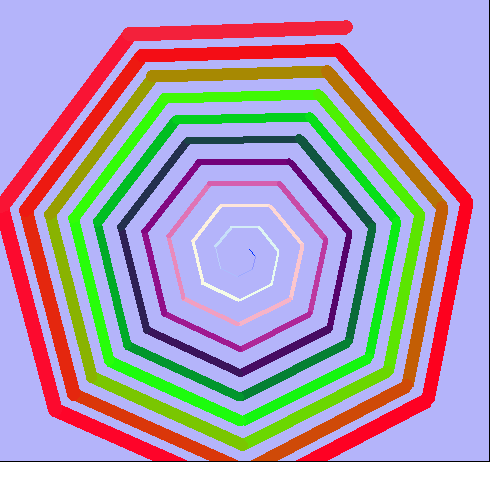# Polyspirals ( a Rosetta Code task.).-One of those graphic exercizes just crying out for clever colour effects..

```
'A Polyspiral is a spiral made of multiple line segments, whereby each segment is
'   larger (or smaller) than the previous one by a given amount.
'   Each segment also changes direction at a given angle.

'The task: animate a series of polyspirals, by drawing a complete spiral then incrementing the angle,
'   and (after clearing the background) drawing the next, and so on.
'   Every spiral will be a frame of the animation.
'   The animation may stop as it goes full circle or continue indefinitely.
'   The given input values may be varied.

nomainwin

WindowWidth  =500
WindowHeight =500

global sScreens: sScreens =0

open "Polyspiral- let me run for a while!!" for graphics_nsb as #wg

#wg "trapclose [quit]"
#wg "down ; fill 180 180 250 ; color darkblue"
#wg "when leftButtonDown saveScreen"

incr        =   0.0

for iteration =0 to 9999

#wg "cls ; fill 180 180 250"
R       = iteration mod 256
G       = 50
B       = 255 -R
'#wg "color "; str\$( R) +" " +str\$( G) +" " +str\$( B)

x1          = 250
y1          = 250

incr    = (incr + 0.2) mod 360

length  = 10
angle   = incr

#wg "size 1"

for i = 1 to 70
x2          = x1 + cos( angle *3.14159265 /180) * length
y2          = y1 + sin( angle *3.14159265 /180) * length
#wg "line "; x1; " "; y1; " "; x2; " "; y2
#wg "size "; int( 1 +i /5)
x1          = x2
y1          = y2
length      = length + 3
angle       = ( angle + incr) mod 360
#wg "color "; rgb\$( i)
next i

scan
'callDLL #kernel32, "Sleep", 200 as long, ret as void

next iteration

wait

function rgb\$( i)
r\$ =str\$( int( 128 +127 *sin( i *0.12)))
g\$ =str\$( int( 128 +127 *sin( i *0.17)))
b\$ =str\$( int( 128 +127 *cos( i *0.06)))
rgb\$ =r\$ +" " +g\$ +" " +b\$
end function

[quit]
close #wg
end

sub saveScreen h\$, x, y
#wg "getbmp scr 1 1 500 500"
bmpsave "scr", "poly" +right\$( "000" +str\$( sScreens), 3) + ".bmp" 'un-rem to save screens
sScreens    = sScreens +1
end sub

```